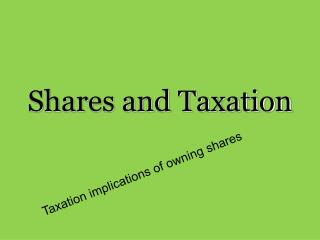DownloadDownload PresentationShares and Taxation

# Shares and Taxation

Download Presentation## Shares and Taxation

- - - - - - - - - - - - - - - - - - - - - - - - - - - E N D - - - - - - - - - - - - - - - - - - - - - - - - - - -
##### Presentation Transcript

1. Shares and Taxation Taxation implications of owning shares

2. Tax on Dividends • Before 1987 a company would pay tax on their profits (dividends) and then you as a shareholder would pay tax on your income – so the Government was kind of getting 2 lots of tax out of one payment. • DIVIDEND IMPUTATION - is a method that gives tax advantages to shareholders who receive dividends. • FULLY FRANKED Dividends have the tax paid on them by the company at the company rate 30%. • This means when you get the dividend tax has already been paid – giving you the possibility of : • not paying tax on the dividends • Paying reduced tax on the dividends • Getting money back in the form of a tax return • Depending on your marginal tax rate

3. This system has benefits for shareholders, making dividend payments more attractive. • If your marginal tax rate is below the company tax rate you will get a refund!

4. Calculating the Imputation Credit • In all these calculations we assume that the Business Tax rate is 30% 0.24 x 1260 302.40 x 30/70 30 is the tax rate

5. Calculate the tax owing and then the after tax return if you had the shares above and had a marginal tax rate of : (a) 17% and (b) 47%. Income declared = Unfranked amount + Franked amount + Imputation Credit Income Declared = 379.5 + 162.64 = 542.14 Tax Liability = Income declared x marginal tax rate Tax Liability = 542.14 x 17% = \$92.16 Tax Owing = Tax liability – Imputation Credit Tax Owing = 92.16 – 162.64 = -70.48 You are owed \$70.48. After Tax Return 379.50 + 70.48 = \$449.48

6. Calculate the tax owing and then the after tax return if you had the shares above and had a marginal tax rate of : (a) 17% and (b) 47%. Income declared = Unfranked amount + Franked amount + Imputation Credit Income Declared = 379.5 + 162.64 = 542.14 Tax Liability = Income declared x marginal tax rate Tax Liability = 542.14 x 47% = \$254.81 Tax Owing = Tax liability – Imputation Credit Tax Owing = 254.81 – 162.64 = 92.17 You are owe \$92.17 After Tax Return 379.50 – 92.17 = \$287.33

7. Calculating the Imputation Credit • In all these calculations we assume that the Business Tax rate is 30% 0.24 x 1260 302.40 x 30/70 30 is the tax rate